Updating search results...

# 11 Results

View
Selected filters:
• MCCRS.Math.Content.7.NS.A.1c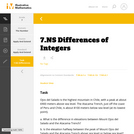Unrestricted Use
CC BY
Rating
0.0 stars

This is a task from the Illustrative Mathematics website that is one part of a complete illustration of the standard to which it is aligned. Each task has at least one solution and some commentary that addresses important aspects of the task and its potential use.

Subject:
Mathematics
Numbers and Operations
Material Type:
Activity/Lab
Provider:
Illustrative Mathematics
Provider Set:
Illustrative Mathematics
Author:
Illustrative Mathematics
08/06/2015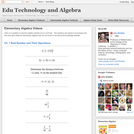Conditional Remix & Share Permitted
CC BY-NC
Rating
0.0 stars

Visually searchable database of Algebra 1 videos. Click on a problem and watch the solution on YouTube. Copy and paste this material into your CMS. Videos accompany the open Elementary Algebra textbook published by Flat World Knowledge.

Subject:
Algebra
Mathematics
Material Type:
Lecture
Lecture Notes
Provider:
Individual Authors
Provider Set:
Individual Authors
Author:
John Redden
04/29/2012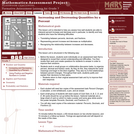Only Sharing Permitted
CC BY-NC-ND
Rating
0.0 stars

This lesson unit is intended to help teachers assess how well students are able to interpret percent increase and decrease, and in particular, to identify and help students who have the following difficulties: translating between percents, decimals, and fractions; representing percent increase and decrease as multiplication; and recognizing the relationship between increases and decreases.

Subject:
Mathematics
Ratios and Proportions
Material Type:
Assessment
Lesson Plan
Provider:
Shell Center for Mathematical Education
Provider Set:
Mathematics Assessment Project (MAP)
04/26/2013Conditional Remix & Share Permitted
CC BY-NC
Rating
0.0 stars

Four full-year digital course, built from the ground up and fully-aligned to the Common Core State Standards, for 7th grade Mathematics. Created using research-based approaches to teaching and learning, the Open Access Common Core Course for Mathematics&nbsp;is designed with student-centered learning in mind, including activities for students to develop valuable 21st century skills and academic mindset.

Subject:
Mathematics
Material Type:
Full Course
Provider:
Pearson
10/06/2016Conditional Remix & Share Permitted
CC BY-NC
Rating
0.0 stars

Working With Rational Numbers

Type of Unit: Concept

Prior Knowledge

Students should be able to:

Compare and order positive and negative numbers and place them on a number line.
Understand the concepts of opposites absolute value.

Lesson Flow

The unit begins with students using a balloon model to informally explore adding and subtracting integers. With the model, adding or removing heat represents adding or subtracting positive integers, and adding or removing weight represents adding or subtracting negative integers.

Students then move from the balloon model to a number line model for adding and subtracting integers, eventually extending the addition and subtraction rules from integers to all rational numbers. Number lines and multiplication patterns are used to find products of rational numbers. The relationship between multiplication and division is used to understand how to divide rational numbers. Properties of addition are briefly reviewed, then used to prove rules for addition, subtraction, multiplication, and division.

This unit includes problems with real-world contexts, formative assessment lessons, and Gallery problems.

Subject:
Algebra
Mathematics
Material Type:
Unit of Study
Provider:
Pearson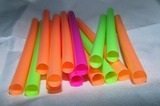Conditional Remix & Share Permitted
CC BY-NC
Rating
0.0 stars

Subject:
Numbers and Operations
Material Type:
Lesson Plan
09/21/2015Conditional Remix & Share Permitted
CC BY-NC
Rating
0.0 stars

Students critique and improve their work on the Self Check, then work on more addition and subtraction problems.Students solve problems that require them to apply their knowledge of adding and subtracting positive and negative numbers.Key ConceptsTo solve the problems in this lesson, students use their knowledge of addition and subtraction with positive and negative numbers.Goals and Learning ObjectivesUse knowledge of addition and subtraction with positive and negative numbers to write problems that meet given criteria.Assess and critique methods for subtracting negative numbers.Find values of variables that satisfy given inequalities.

Subject:
Numbers and Operations
Material Type:
Lesson Plan
09/21/2015Conditional Remix & Share Permitted
CC BY-NC
Rating
0.0 stars

Students find the distance between points on a number line by counting and by using subtraction. They then use subtraction to find differences in temperatures.Students discover that the distance between any two points on the number line is the absolute value of their difference, and apply this idea to solve problems.Key ConceptsStudents know from earlier grades that the distance between two positive numbers on the number line can be found by subtracting the lesser number from the greater number. For example, the distance between 5 and 11 is 11 &ndash; 5, or 6. We can also state the rule for finding distance as &ldquo;The distance between two positive numbers is the absolute value of their difference.&rdquo; With this version of the rule, we don&rsquo;t have to consider which number is greater; the result is the same either way. Using the example of 5 and 11, the distance is |11 &ndash; 5| or |5 &ndash; 11|, both of which are equal to 6.This idea extends to the entire number line, including numbers to the left of 0. That is, the distance between any two numbers is the absolute value of their difference. For example, the distance between &ndash;5 and 3 is |&ndash;5 &ndash; 3| = |&ndash;8| = 8 or |3 &ndash; (&ndash;5)| = |8| = 8, and the distance between &ndash;12 and &ndash;7 is |&ndash;12 &ndash; (&ndash;7)| = |&ndash;5| = 5 or |&ndash;7 &ndash; (&ndash;12)| = |5| = 5.Goals and Learning ObjectivesUnderstand the relationship between the distance between two points on the number line and the difference in the coordinates of those points.Find distances in real-life situations.

Subject:
Numbers and Operations
Material Type:
Lesson Plan
09/21/2015Conditional Remix & Share Permitted
CC BY-NC
Rating
0.0 stars

Students use number lines to solve addition and subtraction problems involving positive and negative fractions and decimals. They then verify that the same rules they found for integers apply to fractions and decimals as well. Finally, they solve some real-world problems.Key ConceptsThe first four lessons of this unit focused on adding and subtracting integers. Using only integers made it easier for students to create models and visualize the addition and subtraction process. In this lesson, those concepts are extended to positive and negative fractions and decimals. Students will see that the number line model and rules work for these numbers as well.Note that rational number will be formally defined in Lesson 15.Goals and Learning ObjectivesExtend models and rules for adding and subtracting integers to positive and negative fractions and decimals.Solve real-world problems involving addition and subtraction of positive and negative fractions and decimals.

Subject:
Numbers and Operations
Material Type:
Lesson Plan
09/21/2015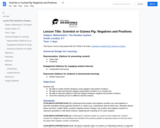Conditional Remix & Share Permitted
CC BY-NC
Rating
0.0 stars

Students explore the concepts of positive and negative numbers through Newton's Third Law and rocket thrust, and other examples.

Subject:
Mathematics
Material Type:
Lesson Plan
Provider:
National Air and Space Museum
Author:
National Air and Space Museum
06/13/2022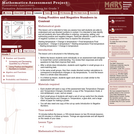Only Sharing Permitted
CC BY-NC-ND
Rating
0.0 stars

This lesson unit is intended to help teachers assess how well students are able to understand and use directed numbers in context. It is intended to help identify and aid students who have difficulties in ordering, comparing, adding, and subtracting positive and negative integers. Particular attention is paid to the use of negative numbers on number lines to explore the structures: starting temperature + change in temperature = final temperature final temperature Đ change in temperature = starting temperature final temperature Đ starting temperature = change in temperature.

Subject:
Mathematics
Numbers and Operations
Material Type:
Assessment
Lesson Plan
Provider:
Shell Center for Mathematical Education
Provider Set:
Mathematics Assessment Project (MAP)